Tributaries

The first tributary fill pool with water in 15 hours.
The second tributary fill pool in 10 hours. For how many hours the pool is filled with both tributaries?

Result

x =  6 h

Solution:

x(1/15+1/10)=1

5x = 30

x = 6

Calculated by our simple equation calculator.

Leave us a comment of example and its solution (i.e. if it is still somewhat unclear...):Be the first to comment!To solve this example are needed these knowledge from mathematics:

Looking for help with calculating harmonic mean? Looking for a statistical calculator? Do you have a linear equation or system of equations and looking for its solution? Or do you have quadratic equation?

Next similar examples:

1. Two tributaries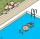Two tributaries of the pool fill it in 10 hours. One of the tributaries would fill 15 hours. How long would fill the first tributary?
2. The tank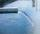The tank had 9 inflows to be filled in 21 days. After 9 days, 3 trips out. How many days did the remaining 6 tributaries fill the tank?
3. Two looms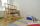On two different looms with different performance can be make required quantity of textile in 6 hours when both work together. On the first machine this quantity of the textile can make in 10 hours. How long should take make same quantity of textile on a s
4. Three peopleThree people start doing a work at a same time. The first worked only 2 hours. The second ended 3 hours before the end. On an individual basis, it would take the first time to do the work 10 hours, second 12 hours and 15 hours third. How many hours did it.
5. Workers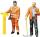Two workers fulfill a certain task when working together for 5 days, the first if he worked himself done work in 13 days. How many days will do whole job a second worker?
6. The factoryThe factory z can complete an order in 12 days, factory p in 18 days. For how many days does an order completed when the first two days work only the factory z and then both factories?
7. PumpingThe tank is filled with a larger pump in 12 hours and with scond pump in 15 hours. For how long does the tank fill when both pumps are switched on at the same time?
8. Collection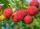John has collected half a bucket of raspberries in 45 minutes, Eva collects the whole bucket in an hour. How many minutes it would take to fill a bucket if the two children work together?
9. 12 Moons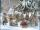Good Marry came to ask twelve moons for help with collecting strawberries. All twelve moons 1200 strawberries picked in 20 minutes. But lazy months July and August cease tear after 5 minutes. How many minutes in total will take collect strawberries?
10. CandyPeter had a sachet of candy. He wanted to share with his friends. If he gave them 30 candies, he would have 62 candies. If he gave them 40 candies, he would miss 8 candies. How many friends did Peter have?
11. Apprentice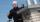Worker and apprentice execute the work for 6 hours. Worker performs himself in 10 hours. How long do the work of an apprentice?
12. Working togetherOne painter paint the nursery in 10 days, the second in 8 days. How many days will the nursery be painted if they work together?
13. Fifth of the numberThe fifth of the number is by 24 less than that number. What is the number?
14. Tonic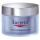One package tonic consume Mom 12 weeks and daughter for 14 weeks. 4 weeks they use the same cream together and then only daughter. How many weeks endured tonic to daughter then?For the construction of the road dam, it is supposed to transport six trucks a total of 18 days. After three days, two more cars were sent to the construction site. How long will it take to carry the material?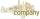Company A would dig a pit for 12 days, company B for 15 days, company C for 20 days and the company D for 24 days. Work began together companies C and D, but after three days joined them other two companies. How long took to dig a pit?Solve the equation: 1/2-2/8 = 1/10; Write the result as a decimal number.